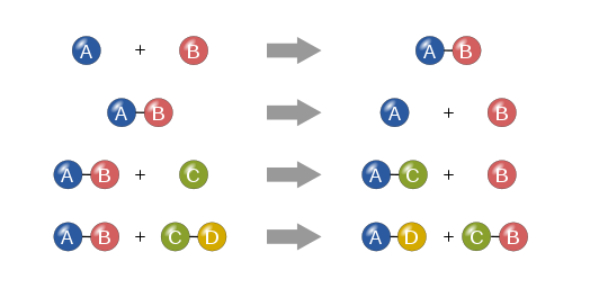# Chemical Reaction Exam: Quiz!

10 Questions | Total Attempts: 49Settings.

• 1.
In reaction A + BC, the rate of reaction can be defined as:
• A.

A decrease of concentration A per time unit

• B.

The multiple concentration of A and B per time unit

• C.

The increase of concentration of A and B per time

• D.

The decrease of concentration C per time unit

• E.

The product of concentration A and B divided by concentration C

• 2.
A reaction is two times faster every 15oC rise in temperature. If the reaction at 30 ° C takes 1 hour, the time required if the reaction is performed at 60 ° C is:
• A.

Two times

• B.

Four time

• C.

Six times

• D.

Eight times

• E.

Ten times

• 3.
Fe (0,2 g) HCl Powder 3 M Powder 2M Bar 3 M Bar 2 M Bar 1 M According to the data above, the fastest reaction occurs in the experiment number:
• A.

1

• B.

2

• C.

3

• D.

4

• E.

5

• 4.
Pay attention to the experimental data form the reaction of A + 3B → 2AB [A] M [B] M V (M/s) 0.01 0.02 0.4 0.02 0.02 0.8 0.03 0.06 10.8 value of total order  reaction is....
• A.

1

• B.

2

• C.

3

• D.

4

• E.

5

• 5.
Pay attention to the experimental data form the reaction of A + 3B → 2AB [A] M [B] M Time (s) 0.02 0.01 32 0.02 0.08 32 0.08 0.04 2 value of contanta rate of reaction are....
• A.

1/8.10-4

• B.

1/32.10-4

• C.

8.10-4

• D.

32.10-4

• E.

1/4.10-4

• 6.
• X + Y → Z
[X] M [Y] M V (M/s) 0.1 0.1 2,2.10-4 0.2 0.3 19,8.10-4 0.1 0.3 19,8.10-4 Graffic oreder reaction that make for X are…
• A.

3

• B.

2

• C.

1

• D.

0

• E.

1/2

• 7.
Reaction : 2NO + Br2 → 2NOBr. Have order reaction : v = k [NO]2[Br2] The above reaction has a total level reaction are:
• A.

3

• B.

2

• C.

1

• D.

0

• E.

1/2

• 8.
For the reaction: A + B → AB get the data: If the concentration of [A] is increased twice at a concentration of B is constant, the reaction rate becomes twice larger. If the concentration of A and B are increased each twice, the reaction rate becomes 8 times larger, the reaction rate equation is…
• A.

V=k(A)2(B)1

• B.

V=k(A)1(B)2

• C.

V=k(A)1(B)1

• D.

V=k(A)1

• E.

V=k(B)2

• 9.
Jika suhu dinaikan 10oC, laju suatu reaksi akan naik dua kali lipat. Jika pada suhu t0C berlangsung 16 menit, pada suhu (t+30)0C reaksinya berlangsung are....
• A.

2 minutes

• B.

2,5 minutes

• C.

1 minutes

• D.

1,5 minutes

• E.

3 minutes

• 10.
• Date of reaction:
A + B → AB2 [A] M [B] M V (M/s) 0.5 0.1 5 0.5 0.4 80 X 0.4 32 1 0.8 640 Determine value of X from above table…
• A.

0,1 M

• B.

0,25 M

• C.

0,2 M

• D.

0,35 M

• E.

0,3 M

Related TopicsBack to top